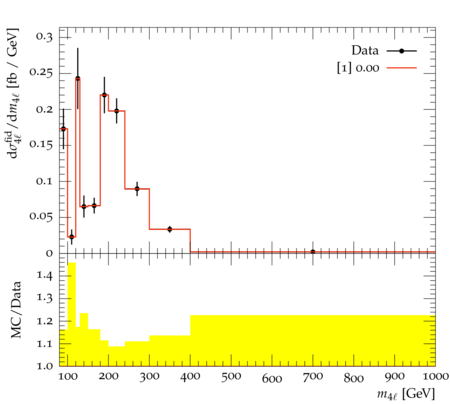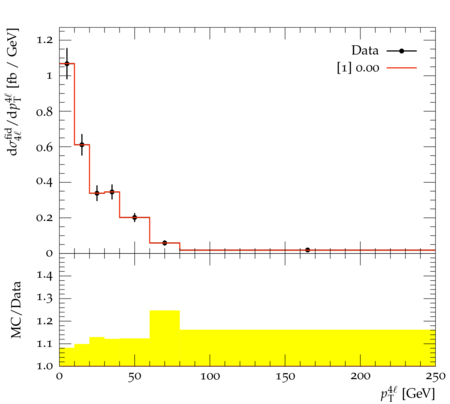### ATLAS_2015_I1394865

Back to index

The four-lepton (4$\ell$, $\ell = e,\mu$) production cross section is measured in the mass range from 80 to 1000 GeV using 20.3 fb$^{-1}$ of data in $pp$ collisions at $\sqrt{s}=8$ TeV collected with the ATLAS detector at the LHC. The $4\ell$ events are produced in the decays of resonant $Z$ and Higgs bosons and the non-resonant $ZZ$ continuum originating from $q\bar{q}$, $gg$, $qg$ initial states. A total of 476 signal candidate events are observed with a background expectation of 26.2$\pm$3.6 events, enabling the measurement of the integrated cross section and the differential cross section as a function of the invariant mass and transverse momentum of the four-lepton system.

ATLAS_2015_I1394865_d01-x01-y01:ATLAS_2015_I1394865_d02-x01-y01:Generated at Sunday, 28. January 2018 12:08PM